Normal view MARC view ISBD view

# Regression modeling with actuarial and financial applications / Edward W. Frees.

Material type:TextSeries: Publisher: Cambridge ; New York : Cambridge University Press, 2010Description: xvii, 565 p. : ill. ; 25 cm.ISBN: 9780521760119; 0521760119; 9780521135962 (pbk.); 0521135966 (pbk.).DDC classification: 519.536 Online resources: WorldCat details | Ebook Fulltext
Contents:
Table of contents 1. Regression and the Normal Distribution -- pt. I. Linear Regression -- 2. Basic Linear Regression -- 3. Multiple Linear Regression -- I -- 4. Multiple Linear Regression -- II -- 5. Variable Selection -- 6. Interpreting Regression Results -- pt. II. Topics in Time Series -- 7. Modeling Trends -- 8. Autocorrelations and Autoregressive Models -- 9. Forecasting and Time Series Models -- 10. Longitudinal and Panel Data Models -- pt. III. Topics in Nonlinear Regression -- 11. Categorical Dependent Variables -- 12. Count Dependent Variables -- 13. Generalized Linear Models -- 14. Survival Models -- 15. Miscellaneous Regression Topics -- pt. IV. Actuarial Applications -- 16. Frequency-Severity Models -- 17. Fat-Tailed Regression Models -- 18. Credibility and Bonus-Malus -- 19. Claims Triangles -- 20. Report Writing: Communicating Data Analysis Results -- 21. Designing Effective Graphs -- Appendix 1. Basic Statistical Inference -- Appendix 2. Matrix Algebra -- Appendix 3. Probability Tables.
Summary: Summary: "Statistical techniques can be used to address new situations. This is important in a rapidly evolving risk management and financial world. Analysts with a strong statistical background understand that a large data set can represent a treasure trove of information to be mined and can yield a strong competitive advantage. This book provides budding actuaries and financial analysts with a foundation in multiple Read more...
Tags from this library: No tags from this library for this title.Average rating: 0.0 (0 votes)
Item type Current location Collection Call number Copy number Status Date due Barcode Item holdsE-Book
E-book
Non-fiction 519.536 FRG 2010 (Browse shelf) Not for loanText
Reserve Section
Non-fiction 519.536 FRG 2010 (Browse shelf) C-1 Not For Loan 27379Text
Circulation Section
Non-fiction 519.536 FRG 2010 (Browse shelf) C-2 Available 27380
Total holds: 0
##### Browsing EWU Library Shelves , Shelving location: Reserve Section Close shelf browser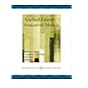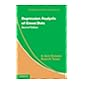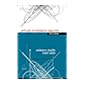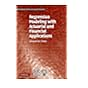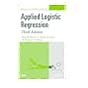519.536 APP 2005 Applied linear statistical models / 519.536 CAR 2013 Regression analysis of count data / 519.536 DRA 2011 Applied regression analysis / 519.536 FRG 2010 Regression modeling with actuarial and financial applications / 519.536 HOA 2013 Applied logistic regression. 519.536 HOA 2013 Applied logistic regression. 519.536 HOA 2013 Applied logistic regression /

Includes bibliographical references and index.

pt. I. Linear Regression --
2. Basic Linear Regression --
3. Multiple Linear Regression --
I --
4. Multiple Linear Regression --
II --
5. Variable Selection --
6. Interpreting Regression Results --
pt. II. Topics in Time Series --
7. Modeling Trends --
8. Autocorrelations and Autoregressive Models --
9. Forecasting and Time Series Models --
10. Longitudinal and Panel Data Models --
pt. III. Topics in Nonlinear Regression --
11. Categorical Dependent Variables --
12. Count Dependent Variables --
13. Generalized Linear Models --
14. Survival Models --
15. Miscellaneous Regression Topics --
pt. IV. Actuarial Applications --
16. Frequency-Severity Models --
17. Fat-Tailed Regression Models --
18. Credibility and Bonus-Malus --
19. Claims Triangles --
20. Report Writing: Communicating Data Analysis Results --
21. Designing Effective Graphs --
Appendix 1. Basic Statistical Inference --
Appendix 2. Matrix Algebra --
Appendix 3. Probability Tables.

Summary:
"Statistical techniques can be used to address new situations. This is important in a rapidly evolving risk management and financial world. Analysts with a strong statistical background understand that a large data set can represent a treasure trove of information to be mined and can yield a strong competitive advantage. This book provides budding actuaries and financial analysts with a foundation in multiple Read more...

Applied Statistics

There are no comments for this item.